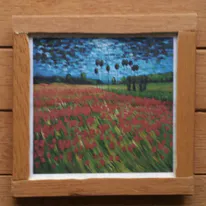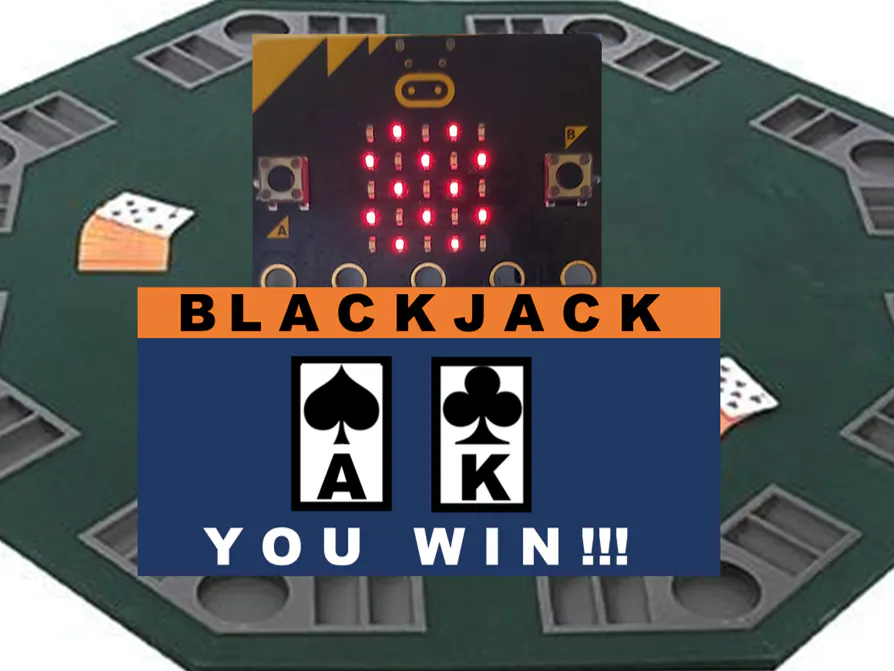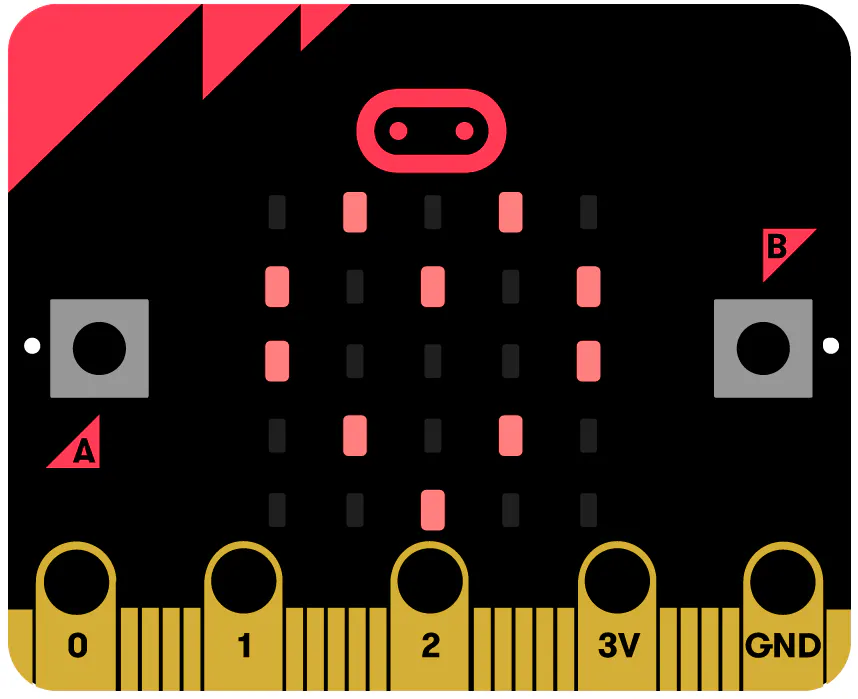# Making card games on micro:bit, Part 1

Learn how to create and shuffle a virtual deck of cards then deal a hand to a player, using a micro:bit. The foundation for any card game.

BeginnerFull instructions provided30 minutes2,242## Things used in this project

### Hardware componentsBBC micro:bit board For Part 1 we just need a BBC micro:bit... but this will be inadequate to display our card game, so we will use additional hardware in Part 2
×1

## Code

JavaScript
MakeCode: https://makecode.microbit.org/_Xq0aAq1RuTxM
Or copy and paste the code below verbatim into the JavaScript window - ignore the 'semi-colon issues'. No extensions are used.
```// TESTING: -----------------------------------------

input.onButtonPressed(Button.A, function () {
for (let index = 0; index <= 4; index++) {
basic.showNumber(playerHand[index])
basic.showString(".")
}
basic.showIcon(IconNames.Yes)
})
input.onButtonPressed(Button.B, function () {
startNewHand()
basic.showNumber(handsPlayed)
basic.showString("X")
basic.showNumber(numberPackShuffles)
basic.showIcon(IconNames.Yes)
})

// Shuffling and resetting deckOfCards -----------------------------------------
//
// very generic - no changes should be required here for different games.
function shufflePack () {
resetPack()
for (let loopX = 0; loopX <= packSize - 1; loopX++) {
cardChosen = randint(0, originPack.length - 1)
deckOfCards.insertAt(loopX, originPack.removeAt(cardChosen))
}
activeCard_PackPosition = 0
numberPackShuffles += 1
}
function getDealtCard () {
if (activeCard_PackPosition >= 52) {
shufflePack()
}
return deckOfCards[activeCard_PackPosition]
}
function resetPack () {
originPack = [packSize]
for (let loopX2 = 0; loopX2 <= packSize - 1; loopX2++) {
originPack[loopX2] = loopX2
}
}

// EDITABLE FUNCTIONS:-----------------------------------------------------------
//
// When you build your card game the functions below will need to be adapted. It
// is likely you will need to implement these functions in some form: they are generic
// to the architecture of any card game.
function startNewHand () {
handsPlayed += 1
playerHandSize = 0
for (let index = 0; index <= 4; index++) {
playerHand[index] = getDealtCard()
activeCard_PackPosition += 1
playerHandSize += 1
}
}
/**
* ------------------------------------------------------------------------------
*/
let playerHandSize = 0
let numberPackShuffles = 0
let activeCard_PackPosition = 0
let originPack: number[] = []
let cardChosen = 0
let playerHand: number[] = []
let deckOfCards: number[] = []
let packSize = 0
let handsPlayed = 0
handsPlayed = 0
let handsWon = 0
packSize = 52
let handMaxSize = 5
deckOfCards = [packSize]
playerHand = [handMaxSize]
shufflePack()
startNewHand()
basic.forever(function () {

})
```

## Credits

### PragmaticPhil

17 projects • 17 followers
Pragmatic hobbyist